PSABACT7 - Grade 8 Mathematics Practice Test 2018

1. (8.EE.5) Line p and line q are shown on this coordinate grid.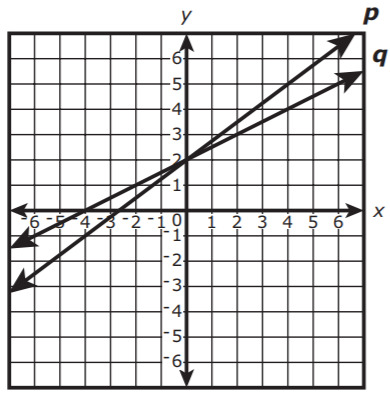Which of the following statements best describes the lines?

Select one:

2. (8.NS.2) Which statement best describes the value of?

Select one:
Part A)

3. (8.G.3) Triangle PQR is shown on the coordinate plane.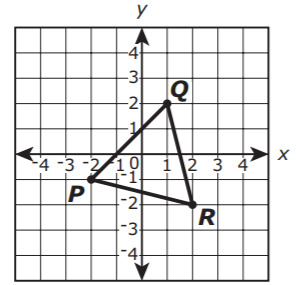Triangle PQR is rotated 90° counterclockwise about the origin to form the image triangle P′Q′R′ (not shown). Then triangle P′Q′R′ is reflected across the x-axis to form triangle P″Q″R″ (not shown).

a. What are the signs of the coordinates (x,y) of point P′?

Select one:
Part B)

b. What are the signs of the coordinates (x,y) of point Q″?

Select one:

4. (8.G.7) The students in a science club planted a rectangular flower garden in front of their school. The garden is 6 feet wide and has a diagonal length of 10 feet.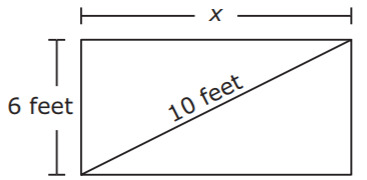What is x, the length in feet of the garden?

Type your answer below as a number (example: 5, 3.1, 4 1/2, or 3/2):
Part A)

5. (8.F.2) Each of these four functions shows a relationship between x and y.

• Function H: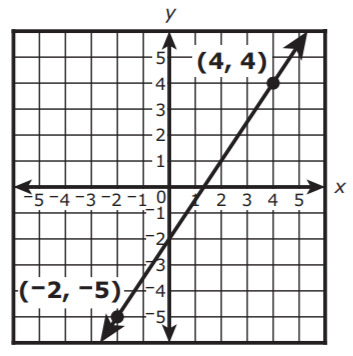• Function I: y = 2.5x + 8
• Function J: Multiply the x value by 3 and subtract 6 to get the y value.
• Function K:   x y -2 4 0 6 2 8 4 10 6 12

a. What is the slope of the line that represents Function H?

Type your answer below as a number (example: 5, 3.1, 4 1/2, or 3/2):
Part B)

a (continued).

Part C)

b. Write an equation in terms of x and y to represent the graph of Function J.

y = _______________

Part D)

c. What is the y-intercept of Function K?

Write the y-intercept in the following format:

(x,y)

with no spaces.

Part E)

c (continued).

Part F)

d. List the four functions in order from the function with the least rate of change to the function with the greatest rate of change.

Type the correct order of each item (eg. 1, 2, 3, etc):

6. (8.EE.5) A solution is 20% bleach. Which graph represents the number of liters of bleach, y, contained in x liters of solution?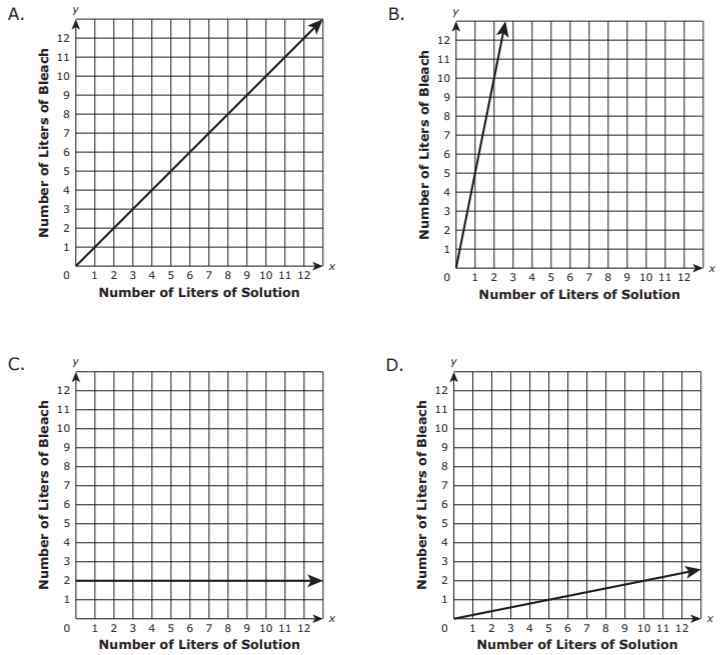Select one:

7. (8.SP.4) A principal surveyed 75 seventh-grade and eighth-grade students. She asked them if they prefer to obtain news from the Internet or to obtain news from television. She created a table to display the data, as shown.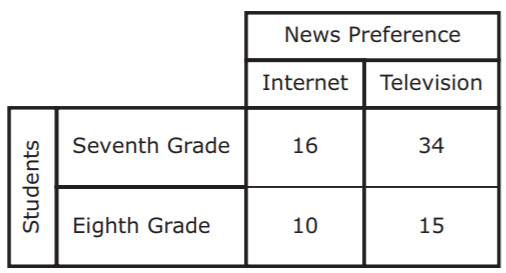Based on the table, select the three correct statements.

Select all that apply:

8. (8.F.5) Mia paddled her canoe from the shore of a lake to an island. She stopped on the island to eat lunch, and then paddled her canoe back to the shore. This graph shows Mia’s distance, in kilometers, from the shore over time.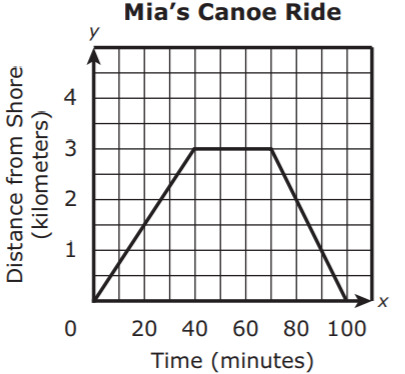Based on the graph, which of the following statements is true?

Select one:

9. (8.SP.2) A scatterplot is shown.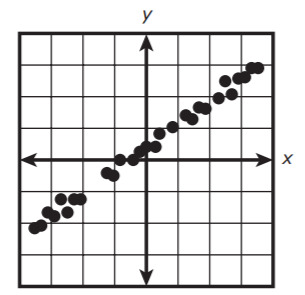Which of the following most closely approximates the line of best fit for the data in the scatterplot?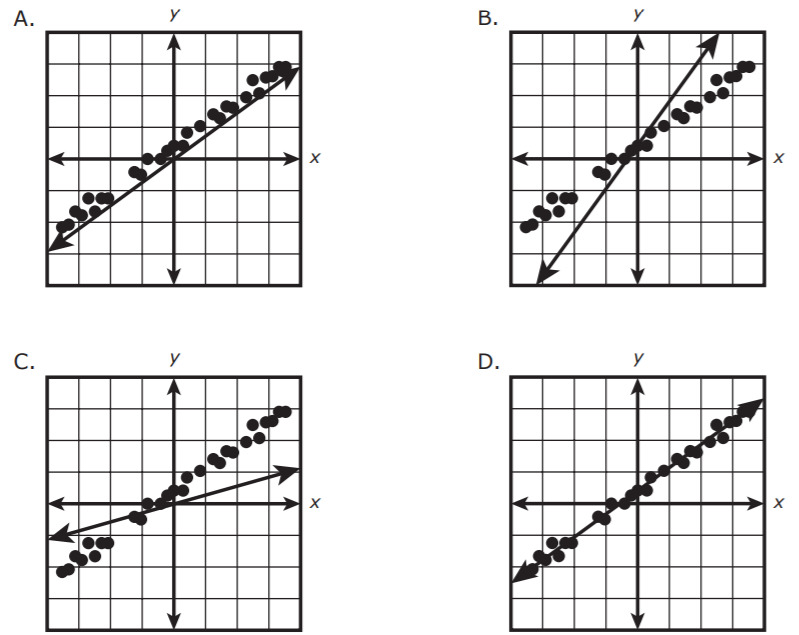Select one: